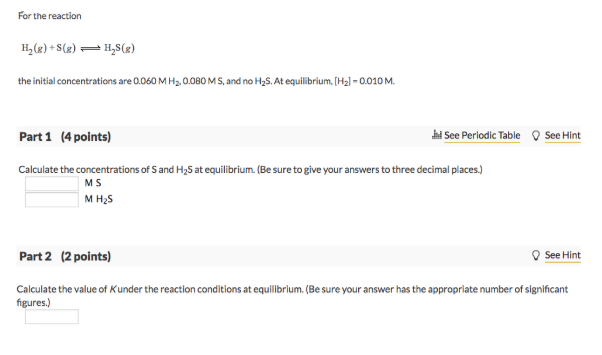# Problem: For the reaction H2 (g) + S (g) ⇌ H2S (g) the initial concentrations are 0.060 M H2, 0.080 MS, and no H2S. At equilibrium, [H] = 0.010 M. Part 1Calculate the concentrations of S and H2S at equilibrium. (Be sure to give your answers to three decimal places.) Part 2Calculate the value of K under the reaction conditions at equilibrium. (Be sure your answer has the appropriate number of significant figures.)

###### FREE Expert Solution
84% (300 ratings)###### Problem Details

For the reaction

H2 (g) + S (g) ⇌ H2S (g)

the initial concentrations are 0.060 M H2, 0.080 MS, and no H2S. At equilibrium, [H] = 0.010 M.

Part 1

Calculate the concentrations of S and H2S at equilibrium. (Be sure to give your answers to three decimal places.)

Part 2

Calculate the value of K under the reaction conditions at equilibrium. (Be sure your answer has the appropriate number of significant figures.)